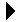Calculator Program# Calculator ProgramI/O With Enumerated Types Download Basic Arrays```--
-- Integer calculator program.  Takes lines of input consisting of
-- <operator> <number>, and applies each one to a display value.  The
-- display value is printed at each step.  The operator is one of =,
-- +, -, *, /, or ^, which correspond to assign, add, subtract, multiply
-- divide, and raise, respectively.  The display value is initially zero.
-- The program terminates on a input of q.
--
with Text_IO;
with Gnat.Io; use Gnat.Io;
procedure Calc is
Op: Character;               -- Operation to perform.
Disp: Integer := 0;          -- Contents of the display.
In_Val: Integer;             -- Input value used to update the display.
begin
loop
-- Print the display.
Put(Disp);
New_Line;

-- Promt the user.
Put("> ");

-- Skip leading blanks and read the operation.
loop
Get(Op);
exit when Op /= ' ';
end loop;

-- Stop when we're s'posed to.
exit when Op = 'Q' or Op = 'q';

-- Read the integer value (skips leading blanks) and discard the
-- remainder of the line.
Get(In_Val);
Text_IO.Skip_Line;

-- Apply the correct operation.
case Op is
when '='      => Disp := In_Val;
when '+'      => Disp := Disp + In_Val;
when '-'      => Disp := Disp - In_Val;
when '*'      => Disp := Disp * In_Val;
when '/'      => Disp := Disp / In_Val;
when '^'      => Disp := Disp ** In_Val;
when '0'..'9' => Put_Line("Please specify an operation.");
when others   => Put_Line("What is " & Op & "?");
end case;
end loop;
end Calc;
```I/O With Enumerated Types Basic Arrays